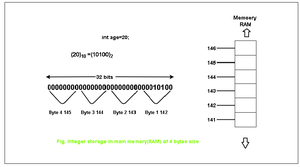Open in App
Not now

# Why integer size varies from computer to computer?

• Difficulty Level : Expert
• Last Updated : 17 Mar, 2023

In a computer, memory is composed of digital memory that stores information binary format, and the lowest unit is called a bit (binary digit). The single-bit denotes a logical value that consists of two states, either o or 1. A bit is a part of the binary number system. Using the combination of these bits, any integer can be represented by the decimal number system.

As digital information is stored in binary bits, computers use a binary number system to represent all numbers such as integers. A byte is a group of 8 bits. In programming languages like C, it is possible to declare variables using the type of that variable, so to store numeric values in computer memory, we use the number of bits internally to represent integers (int).

How Integer Numbers Are Stored In Memory?In the above figure, it can be seen how the integer is stored in the main memory. The above figure gives how the decimal number, i.e., the base 10 number is converted to a binary, i.e., the base 2 number system, and how exactly it is stored in memory.

Why Integer Size Varies From Computer To Computer?

This section focuses on discussing some reasons why integer size varies from computer to computer. Below are the reasons-

1. The aim of C and C++ is to supply in no-time code on all machines. If compilers had to make sure that an int may be an uncommon size for that machine, it might require extra instructions. For nearly all circumstances, that’s not required, all required is that it’s big enough for what the user intends to do with it.
2. Among the benefits of C and C++ is that there are compilers that focus on a huge range of machines, from little 8-bit and 16-bit microcontrollers to large 64-bit multi-core processors. And in fact, some 18, 24, or 36-bit machines too. If some machine features a 36-bit native size, the user would not be very happy if, because some standard says so, you get half the performance in integer math thanks to extra instructions, and can’t use the highest 4 bits of an int.
3. A small microprocessor with 8-bit registers often has support to try 16-bit additions and subtractions (and perhaps also multiplication and divide), but 32-bit math would involve doubling abreast of those instructions and also more work for multiplication and divide. So 16-bit integers (2 bytes) would make far more sense on such a small processor-particularly since memory is perhaps not very large either, so storing 4 bytes for each integer may be a little of a waste.
4. For a 32- or 64-bit machine, the memory range is presumably tons larger, so having larger integers isn’t that much of a drawback, and 32-bit integer operations are an equivalent speed as smaller ones and in some cases “better”- for instance in x86, a 16-bit basic math operation like addition or subtraction requires an additional prefix byte to mention “make this 16-bit”, so math on 16-bit integers would take up more code-space.

This is not universally true, but often true. It is not really useful to extend an int to 64 bits. It wastes space. If required, one can be 64 bits long and still have int be 32 bits. Otherwise, leave only long long for those cases where 64-bit integers are required. Most current implementations do the previous 64 bits long. So there are 16-bit integers (short), 32-bit integers (int), and 64-bit integers (long and long long), all of which are supported by the hardware (in the case of x86), allowing a user to select an appropriate type for every variable. Generally, unless there’s a good reason for the hardware, it is not useful to form kinds larger than their minimum size, since standards-compliant programs can’t expect them to be bigger anyway, and need to work correctly with the minimum size.

Why Int Is Not 16-bits?

They were 32 bits on the 32-bit platforms; the instruction coding for 16-bit operands (on both 32-bit and 64-bit) is one byte longer than that for 32-bit operands. And if a 16-bit value is stored during a register operation, the remainder of the register cannot be used, either on 32-bit or 64-bit, because there’s no instruction coding for “high half 32-bit register”. So 32 bits is kind of a natural size for an operand.

Below is a C++ program to demonstrate the size of an integer in a 64-bit system:

## C++14

 `// C++ program for the above approach` `#include ` `using` `namespace` `std;`   `// Driver Code` `int` `main()` `{` `    ``// sizeof() operator is used to` `    ``// give the size in bytes` `    ``cout << ``sizeof``(``int``);`   `    ``return` `0;` `}`

## Java

 `public` `class` `Main {` `    ``public` `static` `void` `main(String[] args)` `    ``{` `        ``// Sizeof operator is not available in Java` `        ``// Instead we can use the size() method of the` `        ``// IntBuffer class` `        ``System.out.println(Integer.SIZE / ``8``);` `    ``}` `}`

Output:

`4`
Output

`4`

Below is a C++ program to demonstrate the size of an integer in a 32-bit (x86) system:

## C++14

 `// C++ program to implement` `// above approach` `#include ` `using` `namespace` `std;`   `// Driver code` `int` `main()` `{` `    ``// sizeof() operator is used` `    ``// to give the size in bytes` `    ``cout << ``sizeof``(``int``);` `    ``return` `0;` `}`

## Java

 `// Java program to implement` `// above approach`   `import` `java.util.*;`   `public` `class` `Main {` `    ``public` `static` `void` `main(String[] args)` `    ``{` `        ``// sizeof operator is not available in Java` `        ``// Instead, we use the size() method of the` `        ``// primitive wrapper class` `        ``System.out.println(Integer.SIZE / Byte.SIZE);` `    ``}` `}`

Output:

`4`

Output

`2`

1. One of the benefits of varying the size is that fewer CPU cycles are required to read or write.
2. Efficient use of a given architecture depends on 32-bit or 64-bit systems.